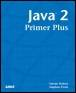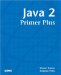# Conversion Between Numeric TypesJava™ 2 Primer PlusBy Steven Haines, Steve Potts Table of Contents Chapter 2.  Keywords, Data Types, and Variables

Variables in Java can be participants in various operations including, but not limited to, addition, subtraction, multiplication, and division. These operations will be discussed in detail in the next chapter, but to complete the discussion of variables you need to know the general form. The general form of a mathematical operation in Java is

` operand operator operand `

Where a variable or a value is an operand and all mathematical operators are included as operators. Consider the following:

` myApples + yourApples `

The result is usually assigned to another variable using the assignment operator (=). The following adds myApples and yourApples and assigns them to the new integer totalApples:

` int totalApples = myApples + yourApples; `

When using numeric variables (variables defined to be ints, floats, and so on) the type does not necessarily have to be the same; in other words you can add an int to a byte. But what happens when you add an int to a byte? Remember that an int is 4 bytes, and a byte is just 1 byte. Take for example a byte with the value 100 and add an int with the value 1000 to it. The result is 1100, but how do we represent that in a byte? There simply are not enough bits to do it!

The answer is to convert the byte to be an int. This is referred to as arithmetic promotion. But what if we add a long, an int, and a byte? Should they all be promoted to a long?

All mathematical operations in Java are performed by promoting each operand in the operation to a specific data type, following these rules in this order:

1. If any of the operands is of type double, the other one will by converted to a double.

2. If any of the operands is of type float, the other one will be converted to a float.

3. If any of the operands is of type long, the other one will be converted to a long.

4. Otherwise all operands will be converted in ints.

The promotion, or conversion, of a narrower type (fewer number of bytes) to a wider type (greater number of bytes), such as the conversion of a short to an int, is accomplished by prepending the narrower type with enough bytes to be the same size as the wider type, and ensuring that the values are identical. For example, converting the byte 10 (written in binary):

` 0000 1010 `

To a short would result in the following:

` 0000 0000 0000 1010 `

Negative value conversions would be a little more complicated because the sign would have to maintained, but Java takes care of that for you.

These rules ensure that all operations resolve to the widest type (in number of bytes) in the operation. Thus, the result of the following operation is a long:

` long l = 10;  int i = 10;  byte b = 10;  long result = l + i + b; `

The int and byte are promoted to be longs before the operation is performed.

#### Conversion Through Assignment

You might have noticed that short and byte variables are promoted to ints during operations, even if there are no ints in the operation. Thus, the following statement would generate an unexpected error:

` byte b1 = 10;  byte b2 = 10;  byte result = b1 + b2; `

The Java compiler would complain about this operation saying that there is a "possible loss of precision" because although b1 and b2 are both bytes, they are promoted to ints before assigning the result to result. A byte has 1 byte, whereas an int has 4 bytes, so assigning an int to a byte loses the top 3 bytes during the assignment. Consider the integer 512, it is represented in binary as

` 0000 0000 0000 0000 0000 0010 0000 0000 `

If you remove the top 3 bytes, you have the following left:

` 0000 0000 `

Which is zero! That is why the compiler disallows the assignment of an int to a byte.

The rule for conversion between primitive numeric types is that a type can be assigned to a wider type (one with more bytes), but not a narrower type (one with less bytes). So, the following conversions are permissible by the compiler:

1. byte short, int, long, float, double

2. short int, long, float, double

3. int long, float, double

4. long float, double

5. float double

6. char int

For example:

` int i = 10;  long l = i; // legal  double d = i; // legal  short s = i; // Illegal! `

 TopJava 2 Primer Plus
ISBN: 0672324156
EAN: 2147483647
Year: 2001
Pages: 332

Similar book on Amazon